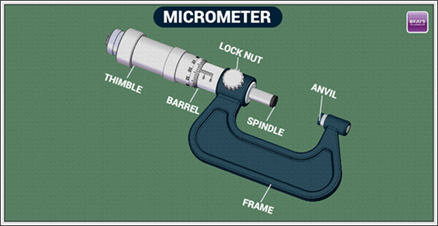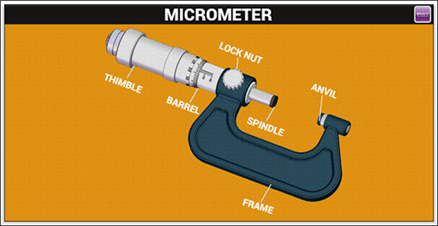# Screw Gauge

For precise measurement of a spherical or a cylindrical object, a screw gauge is the best instrument. An extremely fine-tuned screw gauge can be a bit difficult for untrained hands to use. This article’s objective is to make anyone and everyone aware of the basics of handling a screw gauge.

## Screw Gauge Measurement Using the Micrometer• Get acquainted with the structure of a Screw Gauge: Some sections are movable while others are stationary such as Sleeve, Anvil, Spindle, Thimble Lock, Frame, Thimble, Ratchet Stop.
• Before starting to measure clean the spindle and anvil: Take a paper or a clean cloth and place it in the midst of the spindle and the anvil. Tighten the setup slightly until the paper or cloth is jammed and then pull it out, cleaning the setup in the process. This step is not necessary to do but helps in getting an accurate measurement as preciseness is indispensable.
• Place the object in between the spindle and anvil: Place the object carefully in between the anvil and the spindle. The anvil is stationary in nature, thus place the object against it. The object should be placed properly so that it does not move while taking the measurements.
• The ratchet counter needs to be rotated clockwise to tighten: make sure that the thimbles zero are parallel to the line of the scale on the sleeve.
• Tighten the spindle with the object in the midst: The thimble clicks when being rotated. Apply the amount of force till it clicks three times.
• Lock the thimble while the object is in between: The spindle can be moved even if the thimble is locked. This will help you get the measurement precisely.
• Take the object out cautiously: The screw gauge accuracy can get compromised if it gets scratched or shaken thus make sure to avoid that.
• Before you unlock the spindle, note the reading: Re-measure the whole setup if the single setup loosens.

## Screw Gauge Measurement Through The Inch Micrometer• The thimble has a lot of different scales: The scale measures by one hundred thousand, i.e. one-tenth of an inch or the decimal form is 0.100. Spreads in between those numbers are three lines that are a representation of twenty-five thousandths of an inch, which is represented in decimal would be 0.025. The uniformly spaced lines are a representation of one-thousandth of an inch, when represented in a decimal form it’s written as 0.001. The whole number scale that is represented by the lines on the sleeve is a measure of ten-thousandths of an inch, when represented in the decimal form it’s written as 0.0001.
• The sleeve has whole numbers: The number that’s visible at the end is a representation in thousandths. For instance, if the last number is 6, it would be finally represented as 0.500.
• Note the number of lines after the whole number: Notice the markings next to the 100 thousandth mark and then multiply that by 0.25.
• Notice the marking that’s corresponding to the thimble scale marking (closest to it and beneath the measurement line).
• The three numbers noted down in the earlier process should be noted and added.

## Screw Gauge Formula

There are two parameters used in every screw gauge. They are pitch and least count of a screw gauge.

• Pitch: The pitch of the screw gauge is defined as the distance moved by the spindle per revolution which is measured by moving the head scale over the pitch scale in order to complete one full rotation.
 Pitch of the screw gauge = (distance moved by a screw)/(no. of rotations given)
• Least count: The least count of the screw is defined as the distance moved by the tip of the screw when turned through one division of the head scale.

 Least count (LC) of the screw gauge = (pitch)/(total no.of divisions on the circular scale)
 Least count of micrometer screw gauge = (1 mm)/(100) = 0.01 mm
• Micrometer screw gauge is defined as an instrument that is used for measuring the diameter of thin wires, the thickness of small sheets such as glass or plastics.
 Least count of micrometer = $$\begin{array}{l}\frac{pitch\;of\;screw\;gauge}{total\;number\;of\;divisions\;of\;circular\;scale}\end{array}$$ Least count of $$\begin{array}{l}micrometer = \frac{1mm}{100}= 0.01 mm\end{array}$$

The below video is a quick revision of the screw gauge:## Frequently Asked Questions – FAQs

Q1

### Can a screw gauge measure cylindrical objects?

Yes, it can measure cylindrical objects.
Q2

### What is the formula to calculate the pitch of the screw gauge?

Pitch of the screw gauge = (distance moved by a screw)/(no.of rotations given).

Q3

### Define least count of the screw gauge.

Least count of the screw gauge is the distance moved by the tip of the screw when turned through one division of the head scale.
Q4

### What is a micrometer screw gauge?

It is an instrument that is used for measuring the diameter of thin wires, the thickness of small sheets such as glass or plastics.
Q5

### What is the least count of micrometer?

The least count of micrometer is 0.01mm.
Test your Knowledge on Screw gauge